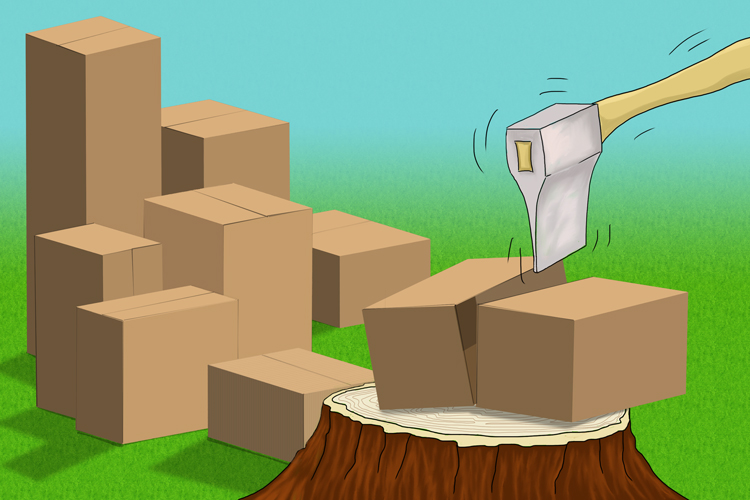# Common core multiplication - box method (grid method)

## Common core multiplication

This method works by splitting each of the numbers into easy multiple sums.Multiply your boxes by splitting them.

If you can remember this for life it will always help tremendously

Example 1

614times5

Split 614 into easier numbers to multiply like 600 and 10 and 4.

Put them into boxes

 600 10 4 5 3000 50 20

For step by step

## Common core multiplication method step by step

Example 1

614times5

 600 10 4 Write down 614 in split form. i.e. 600 and 10 and 4.

 600 10 4 5 Now write down the multiplier

 600 10 4 Multiply 5 into all the top numbers. 5 3000 50 20 5xx600, 5xx10, and 5xx4

 Now add everything up in the boxes 3 0 0 0 5 0 + 2 0 3, 0 7 0

Answer: 615times5=3,070

 3000 50 + 20 3070

Answer: 614times5=3070

Example 2

56times552

Split 56 into easier numbers to multiply like 50 and 6.

Split 552 into easier numbers to multiply like 500 and 50 and 2.

Put them into boxes

 500 50 2 50 25000 2500 100 6 3000 300 12

For step by step

## Common core multiplication method step by step

Example 2

56times552

 500 50 2 Write down 552 in split form. i.e. 500 and 50 and 2.

 500 50 2 50 Now write down 56 in split form. 6

 500 50 2 50 25,000 2,500 100 Multiply 50 into all the top numbers. 6 50xx500, 50xx50, 50xx2

 500 50 2 50 25,000 2,500 100 Multiply 6 into all the top numbers. 6 3,000 30 12 6xx500, 6xx50, 6xx2

 Now add everything up in the boxes 2 5, 0 0 0 3, 0 0 0 2, 5 0 0 3 0 0 + 1 2 3 0, 9 1 2 1

Answer: 56times52=30,912

 25000 3000 2500 300 100 + 12 30,912

Answer: 56times552=30,912

Example 3

27times31

Split 27 into easier numbers to multiply like 20 and 7.

Split 31 into easier numbers to multiply like 30 and 1

Put them into boxes

 20 7 30 600 210 1 20 7

For step by step

## Common core multiplication method step by step

Example 3

27times31

 20 7 Write down 27 in split form. i.e. 20 and 7.

 20 7 30 Now write down 31 in split form. 1

 20 7 30 600 210 Multiply 30 into all the top numbers. 1 30xx20, and 20xx7

 20 7 30 600 210 Multiply 1 into all the top numbers. 1 20 7 1xx20, and 1xx7

 Now add everything up in the boxes 6 0 0 2 1 0 2 0 + 7 8 3 7

Answer: 27times31=837

 600 210 20 + 7 837

Answer: 27times31=837